# NS-CUK Joint Journal Club: S.T.Nguyen, Review on "Neural Sheaf Diffusion: A Topological Perspective on Heterophily and Oversmoothing in GNNs" , NeurIPS 2022

Mar. 31, 2023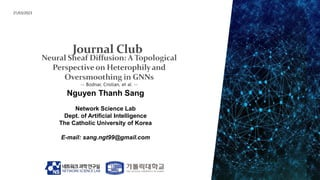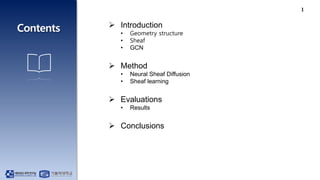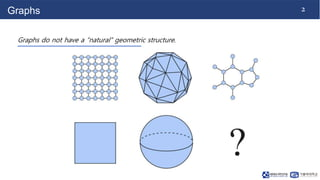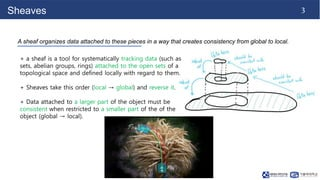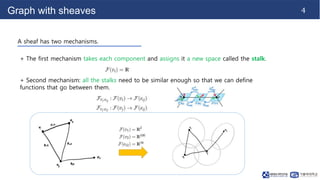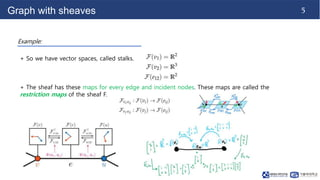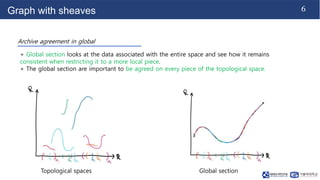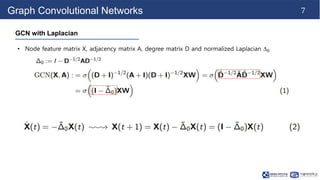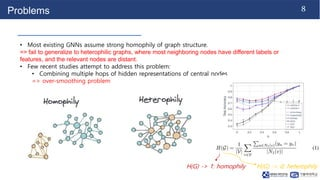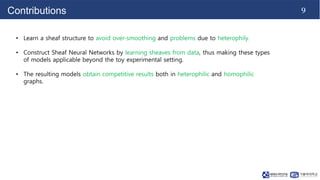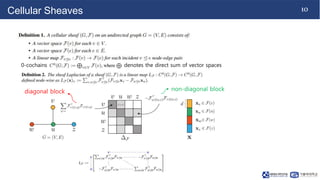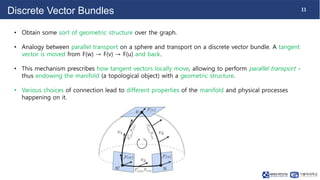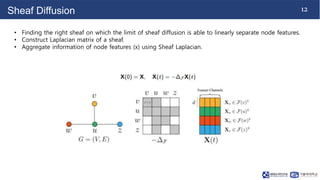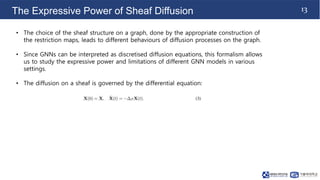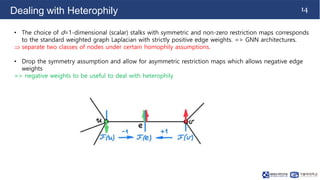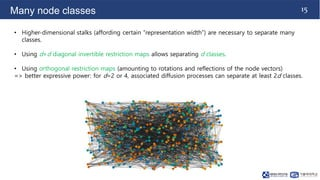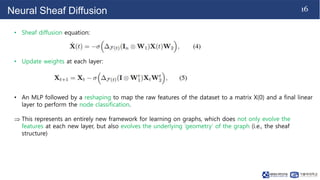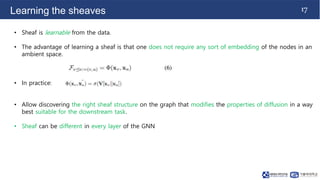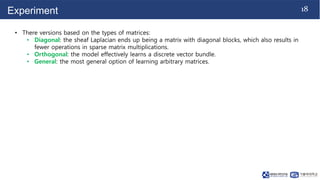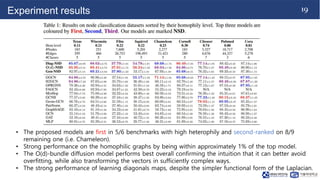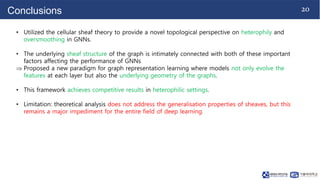1 of 22

### NS-CUK Joint Journal Club: S.T.Nguyen, Review on "Neural Sheaf Diffusion: A Topological Perspective on Heterophily and Oversmoothing in GNNs" , NeurIPS 2022

1. Nguyen Thanh Sang Network Science Lab Dept. of Artificial Intelligence The Catholic University of Korea E-mail: sang.ngt99@gmail.com 21/03/2023
2. 1  Introduction • Geometry structure • Sheaf • GCN  Method • Neural Sheaf Diffusion • Sheaf learning  Evaluations • Results  Conclusions
3. 2 Graphs Graphs do not have a “natural” geometric structure.
4. 3 Sheaves A sheaf organizes data attached to these pieces in a way that creates consistency from global to local. + a sheaf is a tool for systematically tracking data (such as sets, abelian groups, rings) attached to the open sets of a topological space and defined locally with regard to them. + Sheaves take this order (local → global) and reverse it. + Data attached to a larger part of the object must be consistent when restricted to a smaller part of the of the object (global → local).
5. 4 Graph with sheaves A sheaf has two mechanisms. + The first mechanism takes each component and assigns it a new space called the stalk. + Second mechanism: all the stalks need to be similar enough so that we can define functions that go between them.
6. 5 Graph with sheaves Example: + So we have vector spaces, called stalks. + The sheaf has these maps for every edge and incident nodes. These maps are called the restriction maps of the sheaf F.
7. 6 Graph with sheaves Archive agreement in global + Global section looks at the data associated with the entire space and see how it remains consistent when restricting it to a more local piece. + The global section are important to be agreed on every piece of the topological space. Topological spaces Global section
8. 7 Graph Convolutional Networks GCN with Laplacian • Node feature matrix X, adjacency matrix A, degree matrix D and normalized Laplacian ∆0
9. 8 Problems • Most existing GNNs assume strong homophily of graph structure. => fail to generalize to heterophilic graphs, where most neighboring nodes have different labels or features, and the relevant nodes are distant. • Few recent studies attempt to address this problem: • Combining multiple hops of hidden representations of central nodes => over-smoothing problem H(G) -> 1: homophily H(G) -> 0: heterophily
10. 9 Contributions • Learn a sheaf structure to avoid over-smoothing and problems due to heterophily. • Construct Sheaf Neural Networks by learning sheaves from data, thus making these types of models applicable beyond the toy experimental setting. • The resulting models obtain competitive results both in heterophilic and homophilic graphs.
11. 10 Cellular Sheaves diagonal block non-diagonal block 0-cochains denotes the direct sum of vector spaces
12. 11 Discrete Vector Bundles • Obtain some sort of geometric structure over the graph. • Analogy between parallel transport on a sphere and transport on a discrete vector bundle. A tangent vector is moved from F(w) → F(v) → F(u) and back. • This mechanism prescribes how tangent vectors locally move, allowing to perform parallel transport - thus endowing the manifold (a topological object) with a geometric structure. • Various choices of connection lead to different properties of the manifold and physical processes happening on it.
13. 12 Sheaf Diffusion • Finding the right sheaf on which the limit of sheaf diffusion is able to linearly separate node features. • Construct Laplacian matrix of a sheaf. • Aggregate information of node features (x) using Sheaf Laplacian.
14. 13 The Expressive Power of Sheaf Diffusion • The choice of the sheaf structure on a graph, done by the appropriate construction of the restriction maps, leads to different behaviours of diffusion processes on the graph. • Since GNNs can be interpreted as discretised diffusion equations, this formalism allows us to study the expressive power and limitations of different GNN models in various settings. • The diffusion on a sheaf is governed by the differential equation:
15. 14 Dealing with Heterophily • The choice of d=1-dimensional (scalar) stalks with symmetric and non-zero restriction maps corresponds to the standard weighted graph Laplacian with strictly positive edge weights. => GNN architectures.  separate two classes of nodes under certain homophily assumptions. • Drop the symmetry assumption and allow for asymmetric restriction maps which allows negative edge weights => negative weights to be useful to deal with heterophily.
16. 15 Many node classes • Higher-dimensional stalks (affording certain “representation width”) are necessary to separate many classes. • Using d×d diagonal invertible restriction maps allows separating d classes. • Using orthogonal restriction maps (amounting to rotations and reflections of the node vectors) => better expressive power: for d=2 or 4, associated diffusion processes can separate at least 2d classes.
17. 16 Neural Sheaf Diffusion • Sheaf diffusion equation: • Update weights at each layer: • An MLP followed by a reshaping to map the raw features of the dataset to a matrix X(0) and a final linear layer to perform the node classification.  This represents an entirely new framework for learning on graphs, which does not only evolve the features at each new layer, but also evolves the underlying ‘geometry’ of the graph (i.e., the sheaf structure)
18. 17 Learning the sheaves • Sheaf is learnable from the data. • The advantage of learning a sheaf is that one does not require any sort of embedding of the nodes in an ambient space. • In practice: • Allow discovering the right sheaf structure on the graph that modifies the properties of diffusion in a way best suitable for the downstream task. • Sheaf can be different in every layer of the GNN
19. 18 Experiment • There versions based on the types of matrices: • Diagonal: the sheaf Laplacian ends up being a matrix with diagonal blocks, which also results in fewer operations in sparse matrix multiplications. • Orthogonal: the model effectively learns a discrete vector bundle. • General: the most general option of learning arbitrary matrices.
20. 19 Experiment results • The proposed models are first in 5/6 benchmarks with high heterophily and second-ranked on 8/9 remaining one (i.e. Chameleon). • Strong performance on the homophilic graphs by being within approximately 1% of the top model. • The O(d)-bundle diffusion model performs best overall confirming the intuition that it can better avoid overfitting, while also transforming the vectors in sufficiently complex ways. • The strong performance of learning diagonals maps, despite the simpler functional form of the Laplacian.
21. 20 Conclusions • Utilized the cellular sheaf theory to provide a novel topological perspective on heterophily and oversmoothing in GNNs. • The underlying sheaf structure of the graph is intimately connected with both of these important factors affecting the performance of GNNs  Proposed a new paradigm for graph representation learning where models not only evolve the features at each layer but also the underlying geometry of the graphs. • This framework achieves competitive results in heterophilic settings. • Limitation: theoretical analysis does not address the generalisation properties of sheaves, but this remains a major impediment for the entire field of deep learning.
22. 21